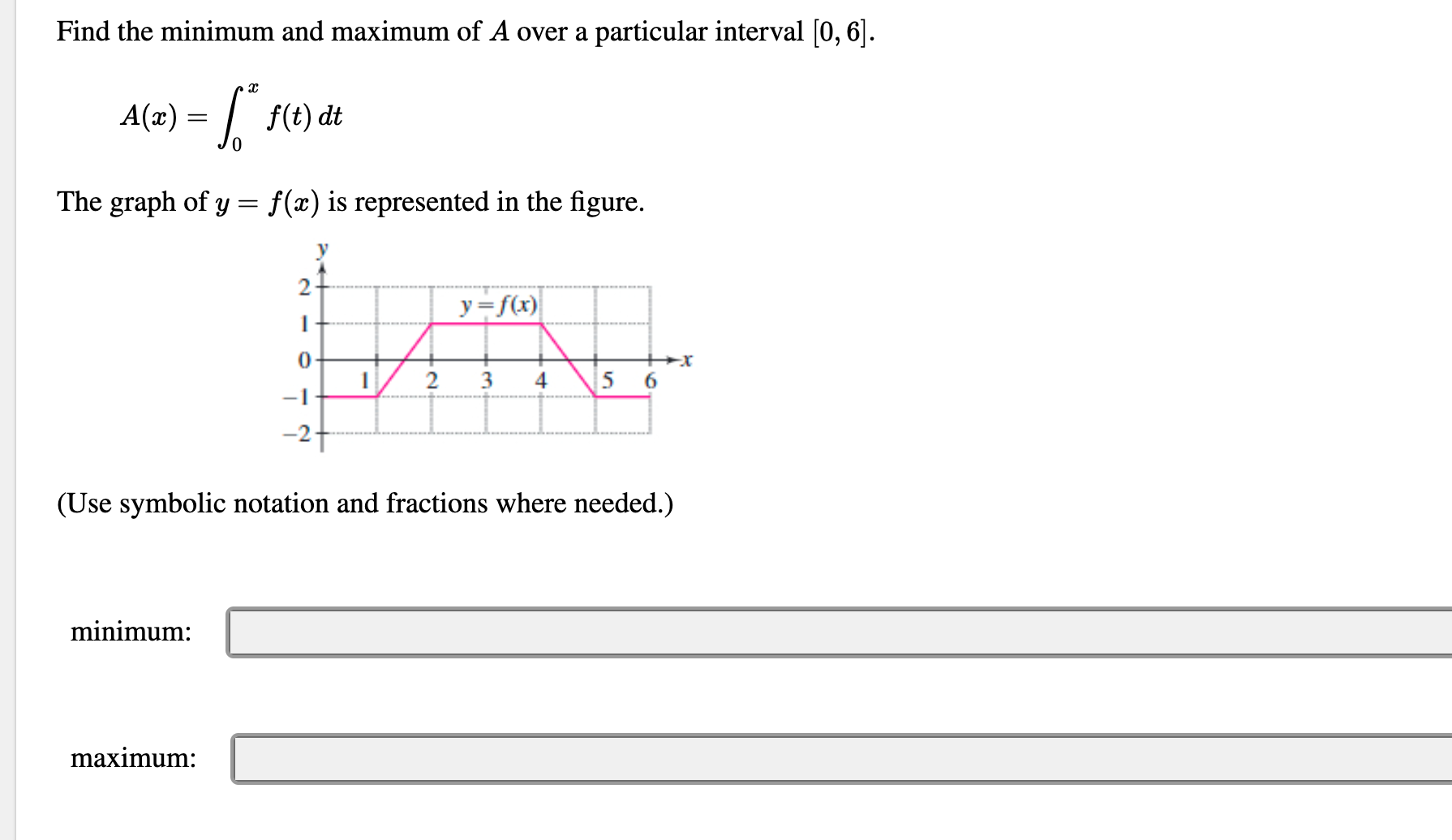# Find the minimum and maximum of A over a particular interval [0, 6].(=) = /,f(t) dtThe graph of y = f(x) is represented in the figure.y=f(x)5 63-1-2+(Use symbolic notation and fractions where needed.)minimum:maximum:

Question
33 viewshelp_outlineImage TranscriptioncloseFind the minimum and maximum of A over a particular interval [0, 6]. (=) = /, f(t) dt The graph of y = f(x) is represented in the figure. y=f(x) 5 6 3 -1 -2+ (Use symbolic notation and fractions where needed.) minimum: maximum: fullscreen
check_circle

Step 1

Find the critical points of A(x). Using A'(x)=0

Step 2

At x=1.5 , A'(x) is going from negative to positive.

Means A(x) is going from decreasing to increasing at x=1.5

So, at x=1.5 there is a local minimum of A(x).

Step 3

At x=4.5 , A'(x) is going from positive to negative

Means A(x) is going from increasing...

### Want to see the full answer?

See Solution

#### Want to see this answer and more?

Solutions are written by subject experts who are available 24/7. Questions are typically answered within 1 hour.*

See Solution
*Response times may vary by subject and question.
Tagged in

### Other